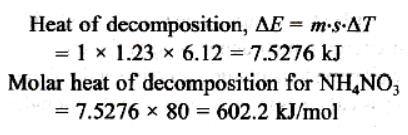Courses

# Test: Thermodynamics And Thermochemistry- 1

## 20 Questions MCQ Test Physical Chemistry | Test: Thermodynamics And Thermochemistry- 1

Description
This mock test of Test: Thermodynamics And Thermochemistry- 1 for Chemistry helps you for every Chemistry entrance exam. This contains 20 Multiple Choice Questions for Chemistry Test: Thermodynamics And Thermochemistry- 1 (mcq) to study with solutions a complete question bank. The solved questions answers in this Test: Thermodynamics And Thermochemistry- 1 quiz give you a good mix of easy questions and tough questions. Chemistry students definitely take this Test: Thermodynamics And Thermochemistry- 1 exercise for a better result in the exam. You can find other Test: Thermodynamics And Thermochemistry- 1 extra questions, long questions & short questions for Chemistry on EduRev as well by searching above.
QUESTION: 1

Solution:
QUESTION: 2

Solution:
QUESTION: 3

### For the isothermal expansion of an ideal gas:

Solution:
QUESTION: 4

In an isochoric process, the increased internal energy is:

Solution:

In an isochoric process, ΔV=0.

Hence, work done PΔV = W = 0.

So, ΔE = q+0.

Hence, the increase in internal energy will be equal to heat absorbed by the system

QUESTION: 5

Internal energy is an example of:

Solution:

Internal energy, enthalpy, and entropy are state quantities because they describe quantitatively an equilibrium state of a thermodynamic system, irrespective of how the system arrived in that state.

QUESTION: 6

The process in which no heat enters or leaves the system is termed as:

Solution:

An adiabatic process occurs without transfer of heat or mass of substances between a thermodynamic system and its surroundings. In an adiabatic process, energy is transferred to the surroundings only as work.

QUESTION: 7

If in a container neither mass and nor heat exchange occurs then it constitutes a

Solution:

An isolated system neither shows exchange of heat nor matter with surroundings.

QUESTION: 8

Which of the following is true for an adiabatic process:

Solution:

A process that does not involve the transfer of heat or matter into or out of a system, so that Q = 0, is called an adiabatic process, and such a system is said to be adiabatically isolated.

QUESTION: 9

Among the following, intensive property is:

Solution:

An intensive property is a property of matter that does not change as the amount of matter changes. It is a bulk property, which means it is a physical property that is not dependent on the size or mass of a sample. In contrast, an extensive property is one that does depend on sample size.

QUESTION: 10

For the reaction of one mole of zinc dust with one mole of sulphuric acid in a bomb calorimeter, ΔU and W correspond to:

Solution:

Bomb calorimeter is commonly used to find the heat of combustion of organic substances which consists of a sealed combustion chamber, called a bomb. If a process is run in a sealed container then no expansion or compression is allowed, so w = 0 and ∆U = q.

∆U < 0, w = 0

QUESTION: 11

Which of the following expressions represent the first law of thermodynamics:

Solution:

The first law of thermodynamics is a version of the law of conservation of energy, adapted for thermodynamic systems. The law of conservation of energy states that the total energy of an isolated system is constant; energy can be transformed from one form to another, but can be neither created nor destroyed. The first law is often formulated

ΔE = Q + W

QUESTION: 12

At 270C one mole of an ideal gas is compressed isothermally and reversibly from a pressure of 2 atm to 10 atm. The value of ΔE and q are (R = 2):

Solution:

Isothermally (at constant temperature) and reversible work.

w = -2.303nrt log(P1/P2)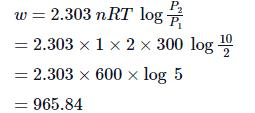at constant temperature , ΔE = 0
ΔE = Q + W,

Q = −W = −965.84 cal

QUESTION: 13

The heat required to raise the temperature of a body by 1K is called:

Solution:
QUESTION: 14

Which of the following is true for the reaction: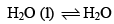at 1000C at one atmosphere:

Solution:
QUESTION: 15

Identify the correct statement regarding entropy:

Solution:
QUESTION: 16

Maximum entropy will be in which of the following:

Solution:

Entropy of gases is highest.

QUESTION: 17

If enthalpies of formation C2H4 (g), CO2 (g) and H2O (l) at 2500C and 1 atm. pressure be 52, –394 and –286 KJ mol–1 respectively. The enthalpy of combustion of C2H4 (g) will be:

Solution: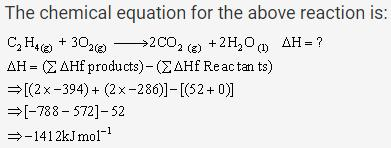QUESTION: 18

Heat of neutralization of strong acid and weak base is:

Solution:
QUESTION: 19

The heat evolved in the combustion of methane is given by the following equations:

CH4 (g) + 2O2 (g) -> CO2 (g) + H2O (l)         ΔH = -890.3 KJ

How many grams of methane would be required to produce 444.15 KJ of heat of combustion:

Solution: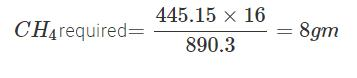QUESTION: 20

One gram sample of NH4NO3 is decomposed in a bomb calorimeter. The temperature of the calorimeter increases by 6.12 K. The heat capacity of the system is 1.23 kJ/g/deg. What is the molar heat of decomposition for NH4NO3?

Solution: